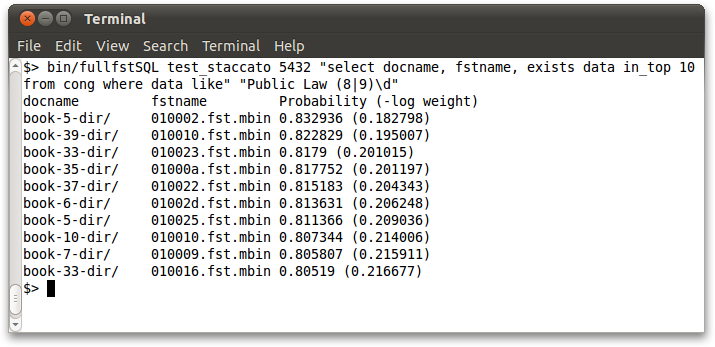# Staccato Usage

We now explain the usage of the programs available under Staccato. After installing Staccato, a number of binaries are available under the bin/ folder. They are of three categories:

## 1. Front-Ends

• fullFSTSQL

Issue a SQL query with a LIKE predicate over the FullFST data and obtain a ranked list of answers. Usage:

```bin/fullFSTSQL <db name> <port number> <SQL query and regex: "select <aux cols>, exists <data col> in_top <num ans> from <table> where <data col> like" "<regex>"> -v (optional, verbose)
```

The first two arguments mention the database and the port to connect to. Then the SQL query and the regex to match the data are specified. The current release has some restrictions on the kind of query and regex that can be issued.[Note 1] The computed answer set is printed on the screen. Example screenshot:• kmapSQL

Similarly, issue the query over the k-MAP data to obtain answers. Usage:

```bin/kmapSQL <db name> <port number> <SQL query and regex: "select <aux cols>, exists <data col> in_top <num ans> from <table> where <data col> like" "<regex>"> -k <k value> -v (optional, verbose)
```

The usage is similar to fullFSTSQL, but the value of k needs to be specified additionally.

• staccatoSQL

Issue the query over the Staccato data to obtain answers. Usage:

```bin/staccatoSQL <db name> <port number> <SQL query and regex: "select <aux cols>, exists <data col> in_top <num ans> from <table> where <data col> like" "<regex>"> -k <k value> -m <m value> -v (optional, verbose)
```

The usage is similar to kmapSQL, but the value of m needs to be specified additionally.

• staccatoSQLIndex

Issue the query over the Staccato data to obtain answers making use of the Staccato inverted index. Usage:

```bin/staccatoSQLIndex <db name> <port number> <SQL query and regex: "select <aux cols>, exists <data col> in_top <num ans> from <table> where <data col> like" "<regex>"> -k <k value> -m <m value> -i <index term> -l <(optional) length of fixed-length regex> -v (optional, verbose)
```

The usage is similar to staccatoSQL, but additionally, the term to look up in the index needs to be specified. Optionally, the length of the regex (if it is fixed length) can be specified.[Note 2]

## 2. Extract/Transform Utilities

• fstmbin

Converts an OCR FST output by OCRopus from the OpenFST format (.fst) to a custom format (.mbin) that includes additional information (or vice versa). The .mbin format is used by all Staccato utilities. Usage:

```bin/fstmbin <.fst file> <.mbin file> <flag: 0 for fst to mbin | 1 for mbin to fst>
```
• kmapgen

Generates a plaintext file with the k-MAP data obtained from an FST. Usage:

```bin/kmapgen <.mbin file> <numPaths> <.kmap file>
```

The second argument is the k value.

• approximation

Generates a plaintext file with the Staccato-approximated data obtained from an FST. Usage:

```bin/approximation <.mbin file> <.stac file> -k <k value> -m <m value> -v (optional, verbose)
```

Both the k and m need to be specified. Verbose mode is optional.

• graphgen

Generates a binary file of the Staccato graph from a .stac file. Usage:

```bin/graphgen <.stac file> <.graph file>
```
• indexgen

Generates a plaintext file with the Staccato index using the given dictionary on the .stac file. Usage:

```bin/indexgen <dict preftrie .txt file> <# states in preftrie> <.stac file> <.index file>
```

The first argument is the textual output of the FST produced by OpenFST after determinizing the dictionary FST, which yields a prefix trie. The second argument is the number of states in that determinized FST. A sample dictionary and the .txt file of its determinized prefix trie FST (with 143664 states) are available in the src/indexing/ folder.

Loads the given FST (in .mbin format) with relevant metadata into the FullFST table. Usage:

```bin/loadfullfst <dbname> <port number> <fullfst table> <docname> <mbinname> <.mbin full filepath>
```

Here, docname is the identifier for the document (or page) and mbinname is the identifier for the FST (typically within that page). These should be the same as used in the master table (refer to the schema details in the Tech Report or from the datasets available for download). Note that the last argument should give the absolute filepath for the .mbin file to be loaded.

Similarly, loads the given k-MAP data (in .kmap format) with relevant metadata into the k-MAP table. Usage:

```bin/loadkmap <dbname> <port number> <kmap table> <docname> <mbinname> <.kmap full filepath>
```

Loads the given Staccato data (in .stac format) with relevant metadata into the Staccato Data table. Usage:

```bin/loadstaccato <dbname> <port number> <staccatodata table> <docname> <mbinname> <.stac file>
```

Loads the given Staccato graph (in .graph format) with relevant metadata into the Staccato Graph table. Usage:

```bin/loadgraph <dbname> <port number> <staccatograph table> <docname> <mbinname> <.graph full filepath>
```
```bin/loadindex <dbname> <port number> <index table> <docname> <mbinname> <.index file>